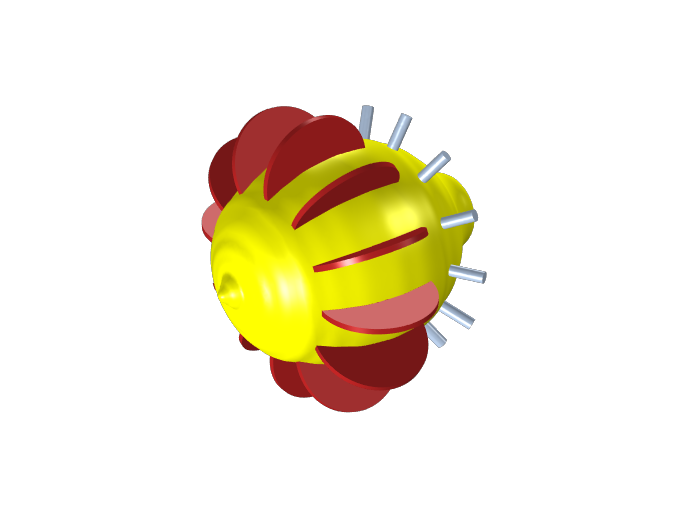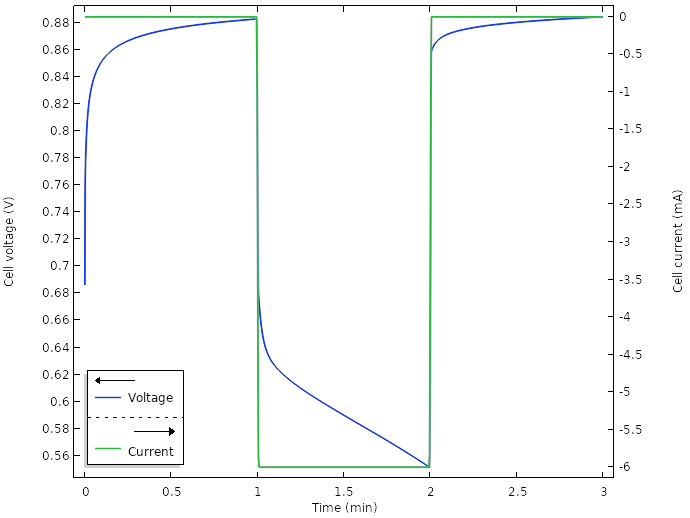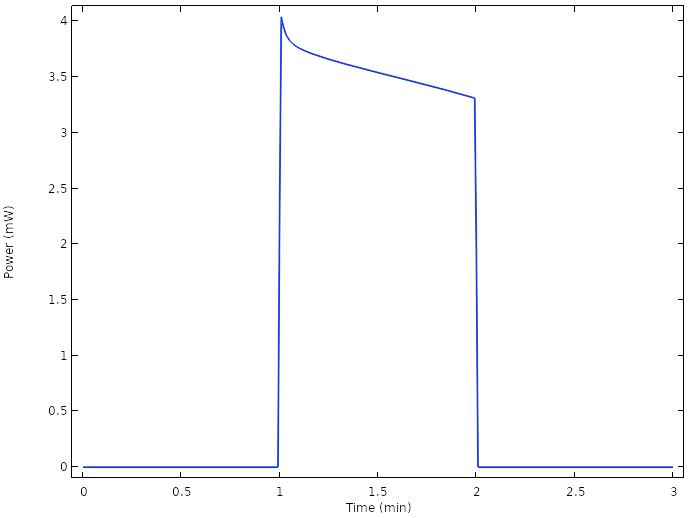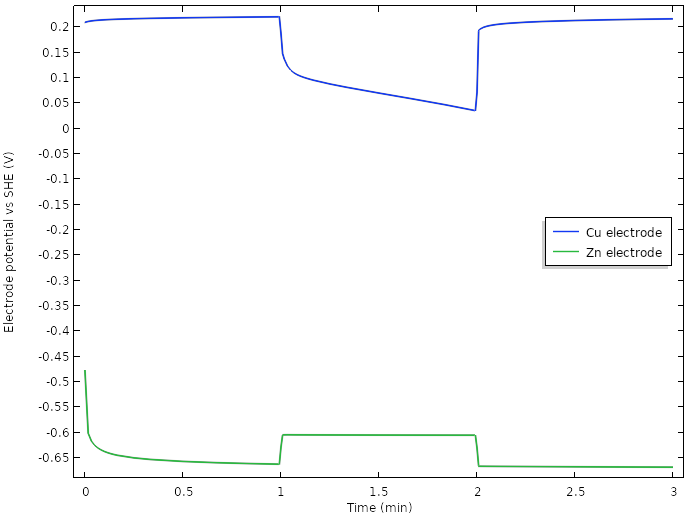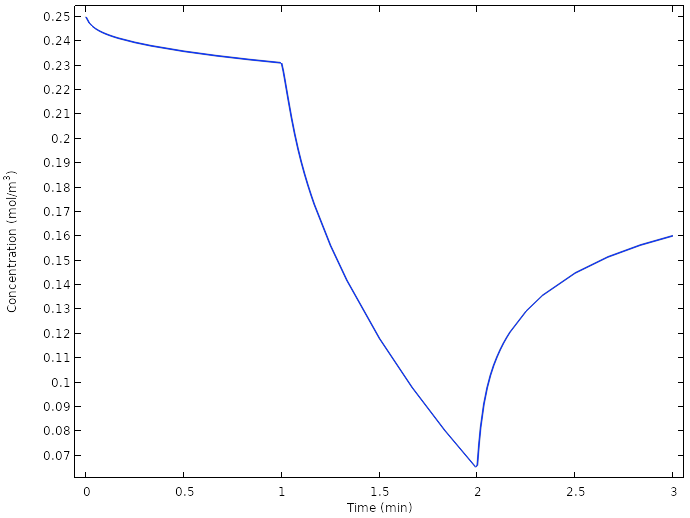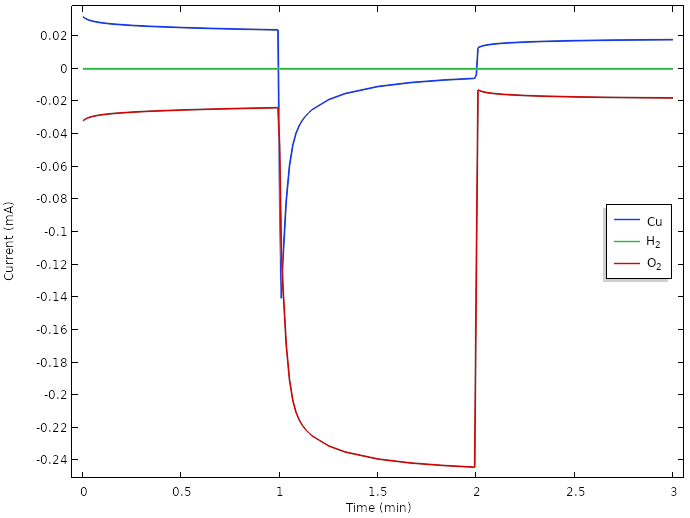# 如何建立电化学模型——以柠檬电池为例

2020年 5月 5日

### 柠檬电池简介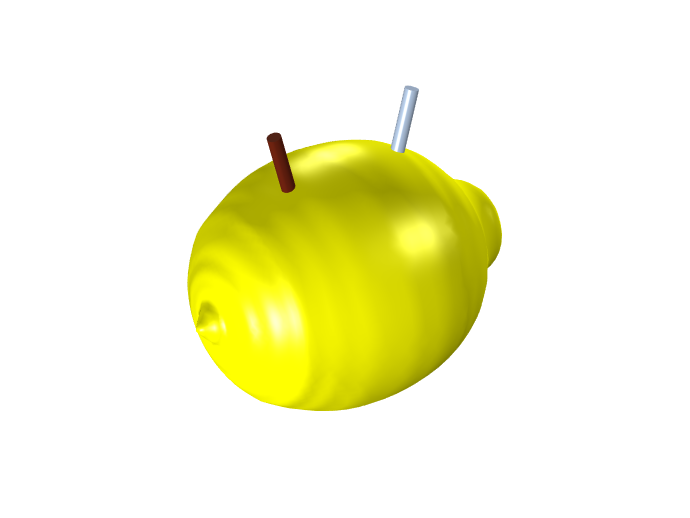### 化学物质和反应

\mathrm{Cu}^{2+}+2e^- \Leftrightarrow \mathrm{Cu(s)} \qquad E_\mathrm{eq}=0.19\mathrm{\;V\;vs\;SHE}
\mathrm{Zn}^{2+}+2e^- \Leftrightarrow \mathrm{Zn(s)} \qquad E_\mathrm{eq}=-0.98\mathrm{\;V\;vs\;SHE}

\mathrm{H}_{2}\mathrm{O} \Leftrightarrow \mathrm{H}^+ + \mathrm{OH}^-

\mathrm{O}_{2}+4\mathrm{H}^{+} + 4e^- \Leftrightarrow 2\mathrm{H}_{2}\mathrm{O} \qquad E_\mathrm{eq}=1.11\mathrm{\;V\;vs\;SHE}
2\mathrm{H}^{+} + 2e^- \Leftrightarrow \mathrm{H}_{2} \qquad E_\mathrm{eq}=-0.12\mathrm{\;V\;vs\;SHE}

### 电解液传输

Zn(s) 和 Cu(s) 的化学活度为常数 1，无需进一步处理。我们还假设电极的形状不会由于金属的溶解而改变。

### 系统边界和几何选择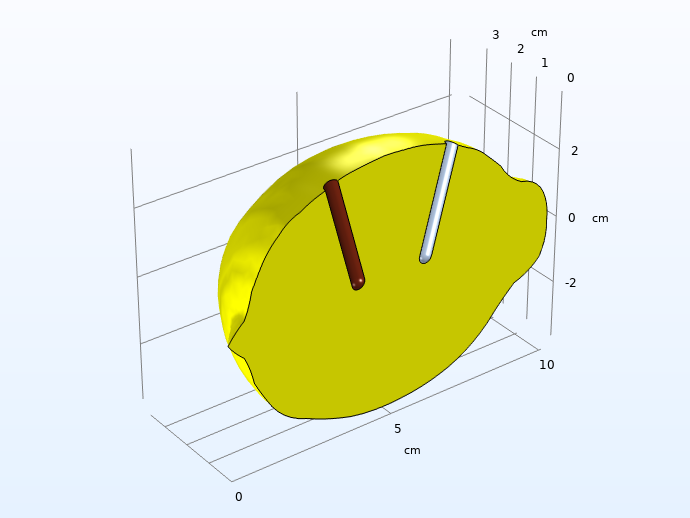### 解释电池运行期间的电压曲线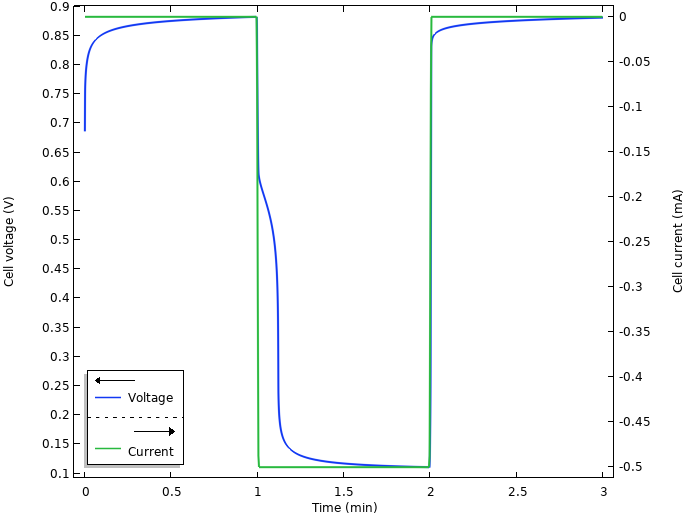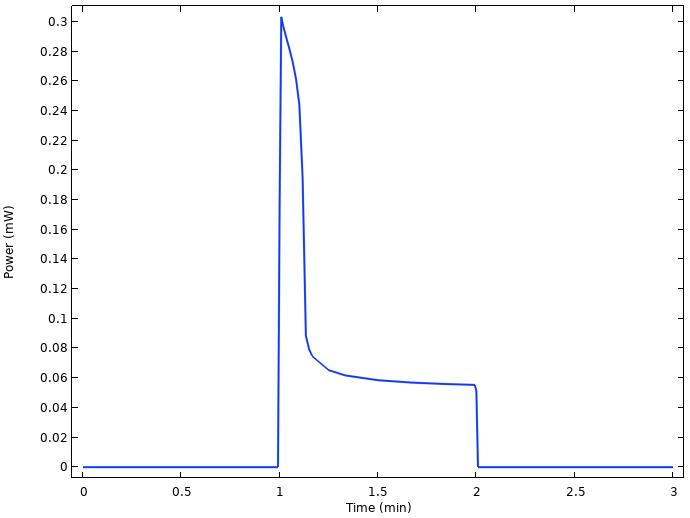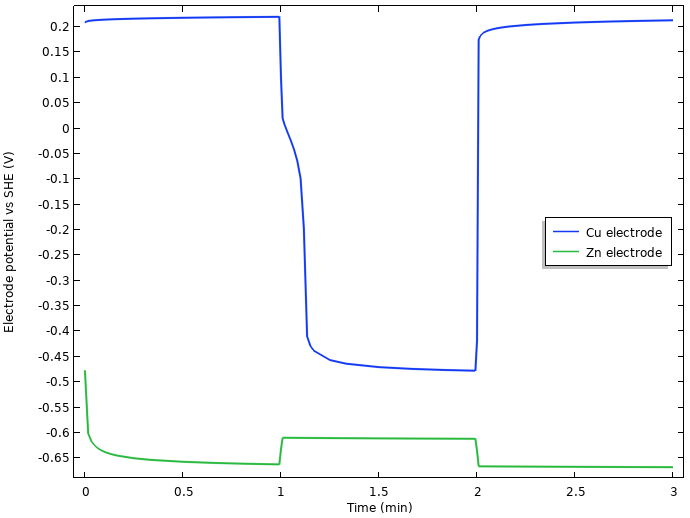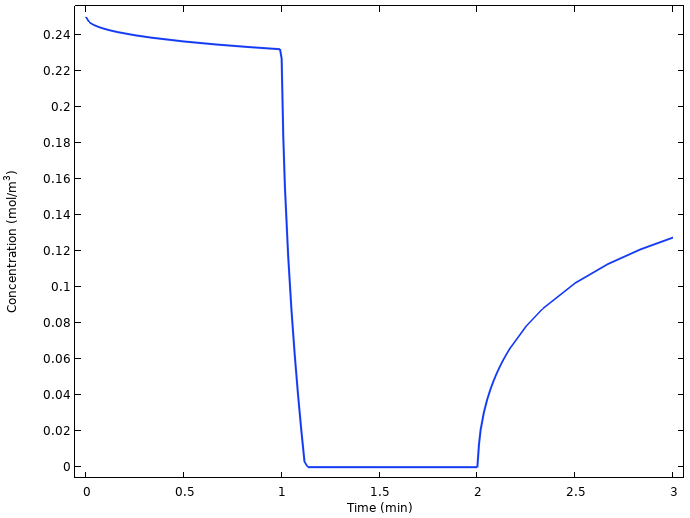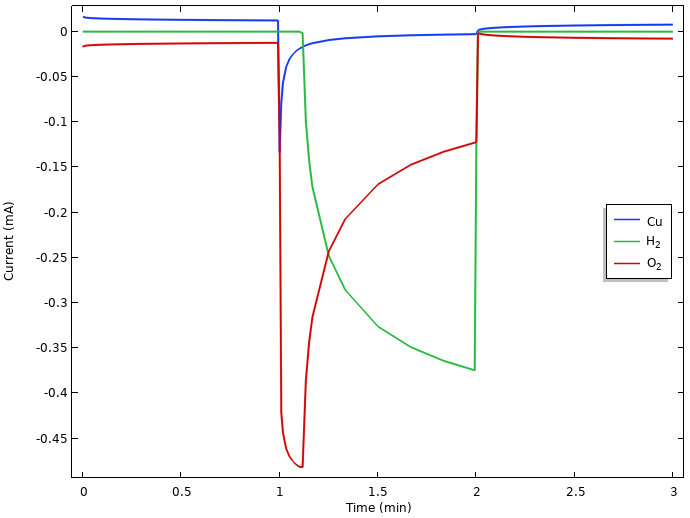### 负极自放电和电池容量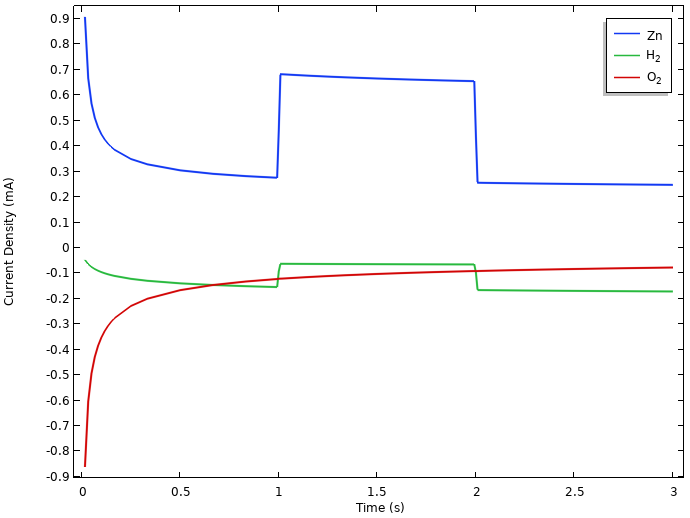### 评估电压损失

1. 电解液中的欧姆压降
2. 驱动锌在负极上溶解的活化过电位
3. 驱动正极上析氢反应（或氧还原）的活化过电位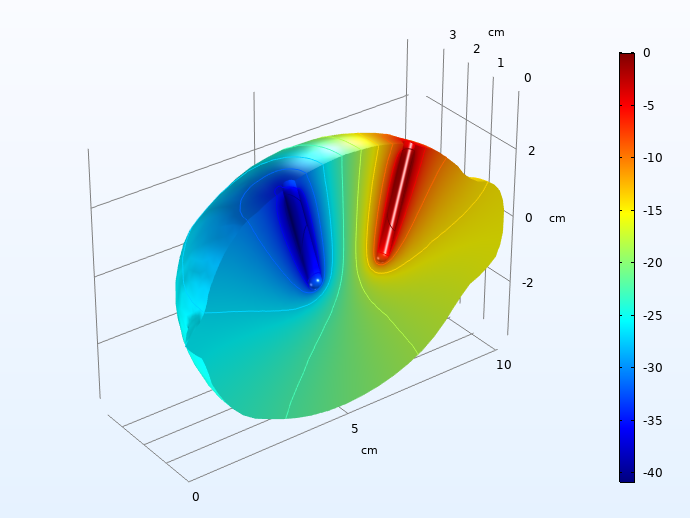40 mV 的电压降很明显，但并不大。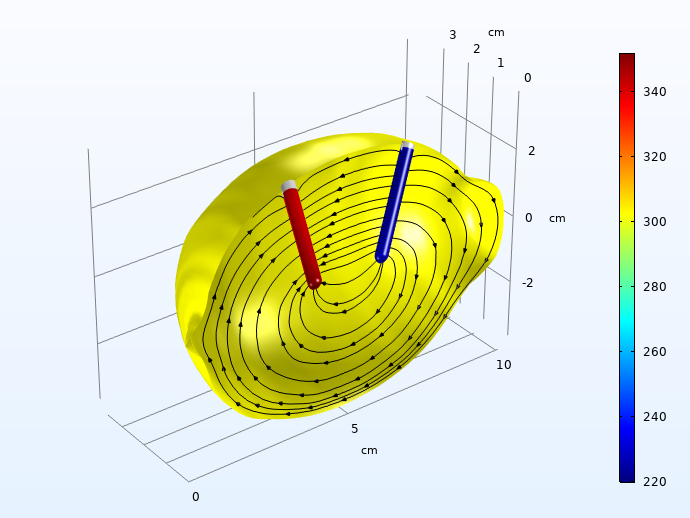### 提高功率输出